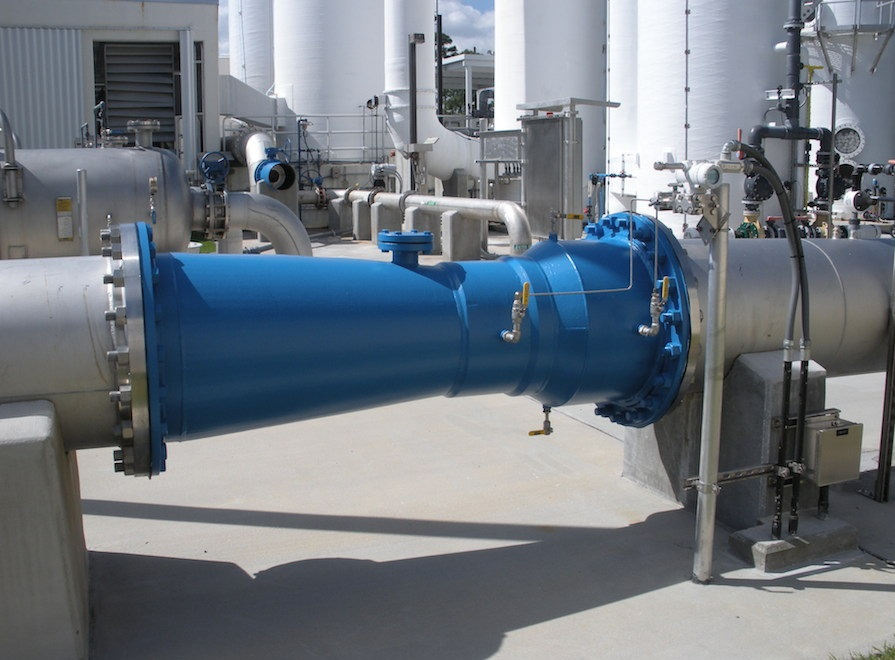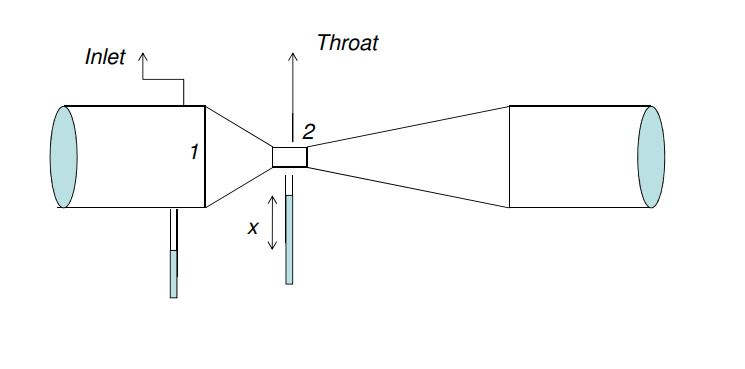# How to use VENTURI METER for Measuring Flow Rate ?Accurate measurement of flow rate of liquids and gases is an essential requirement for maintaining the quality of industrial processes. In fact, most of the industrial control loops control the flow rates of incoming liquids or gases in order to achieve the control objective. As a result, accurate measurement of flow rate is very important. Needless to say that there could be diverse requirements of flow measurement, depending upon the situation.

It could be volumetric or mass flow rate, the medium could be gas or liquid, the measurement could be intrusive or nonintrusive, and so on. As a result there are different types of flow measuring techniques that are used in industries. The common types of flowmeters that find industrial applications can be listed as below:
• The Venturimeter
• The Pitot Tube
• Free Jets
• Orifice Plate or Orifice Meter
• The Flow Nozzles
In these article we will learn about how to use VENTURI METER for Measuring Flow Rate

Basic Principle of Venturi Meter :
When a venture meter is placed in a pipe carrying the fluid whose flow rate is to be measured, a pressure drop occurs between the entrance and throat of the venturimeter. This pressure drop is measured using a differential pressure sensor and when calibrated this pressure drop becomes a measure of flow rate.

How does Venturimeter works ?
Venturimeter is a device used for measuring the rate of flow of a fluid flowing through a pipe. It consists of three parts:1. A short converging part
2. Throat
3. Diverging part

The converging inlet section decreases the area of the fluid stream, causing the velocity to increase and the pressure to decrease. At the centre of the cylindrical throat, the pressure will be at its lowest value, where neither the pressure nor the velocity will be changing. As the fluid enters the diverging section, the pressure is largely recovered lowering the velocity of the fluid. The major disadvantages of this type of flow detection are the high initial costs for installation and difficulty in installation and inspection.

The Venturi effect is the reduction in fluid pressure that results when a fluid flows through a constricted section of pipe. The fluid velocity must increase through the constriction to satisfy the equation of continuity, while its pressure must decrease due to conservation of energy: the gain in kinetic energy is balanced by a drop in pressure or a pressure gradient force. An equation for the drop in pressure due to Venturi effect may be derived from a combination of Bernoulli’s principle and the equation of continuity.

Equation :-Application:
• It is used where high pressure recovery is required.
• Can be used for measuring flow rates of water,gases,suspended solids, slurries and dirty liquids.
• Can be used to measure high flow rates in pipes having diameters in a few meters.

• Less changes of getting clogged with sediments
• Coefficient of discharge is high.
• Its behaviour can be predicted perfectly.
• Can be installed vertically, horizontally or inclinded.

Limitations :-
• They are large in size and hence where space is limited, they cannot be used.
• Expensive initial cost, installation and maintenance.
• Require long laying length. That is, the veturimeter has ti be proceeded by a straight pipe which is free from fittings and misalignments to avoid turbulence in flow, for satisfactory operation. Therefore, straightening vanes are a must.
• Cannot be used in pipes below 7.5cm diameter